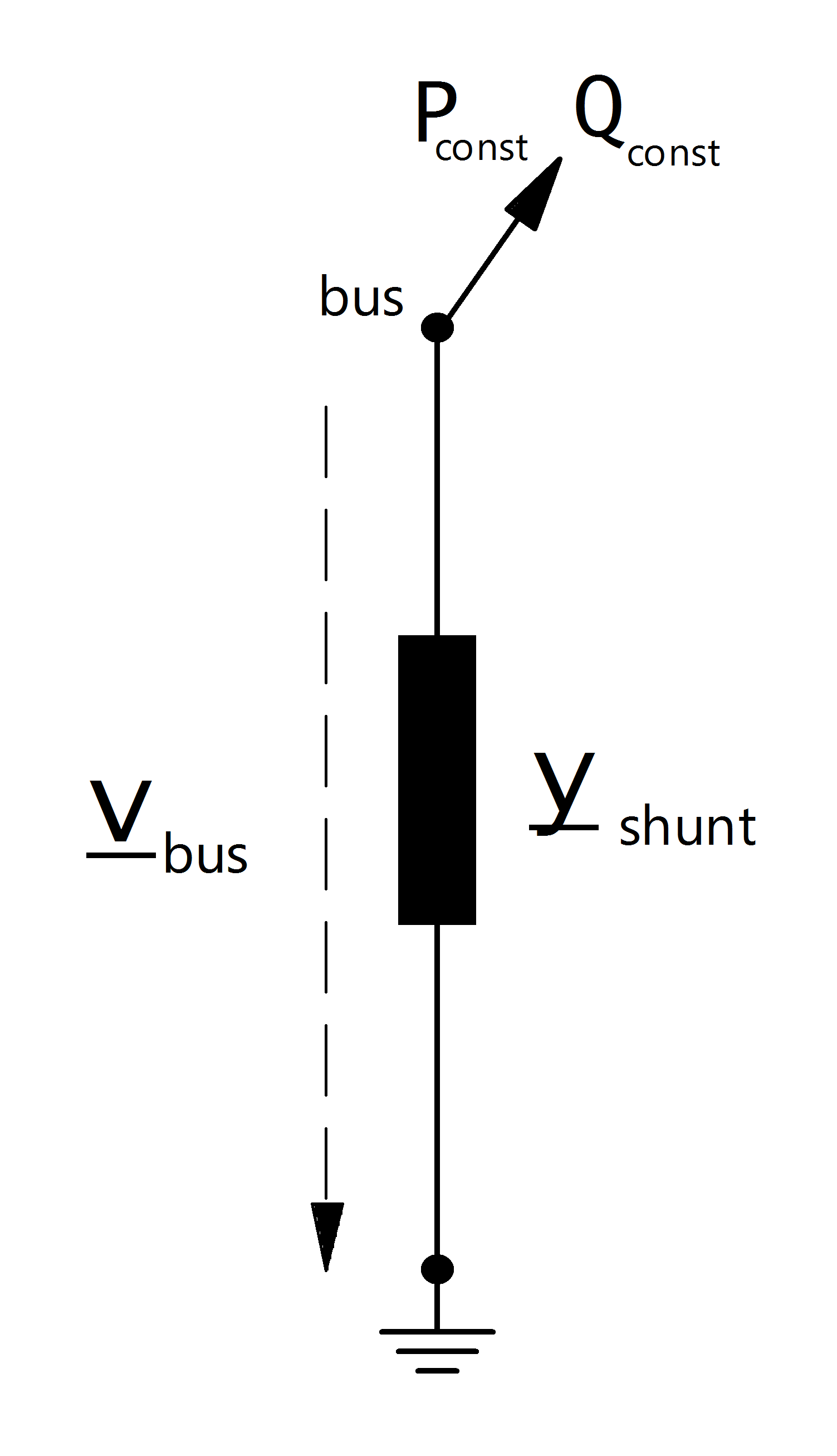# Ward¶

## Create Function¶

pandapower.create_ward(net, bus, ps_mw, qs_mvar, pz_mw, qz_mvar, name=None, in_service=True, index=None)

Creates a ward equivalent.

A ward equivalent is a combination of an impedance load and a PQ load.

INPUT:

net (pandapowernet) - The pandapower net within the element should be created

bus (int) - bus of the ward equivalent

ps_mw (float) - active power of the PQ load

qs_mvar (float) - reactive power of the PQ load

pz_mw (float) - active power of the impedance load in MW at 1.pu voltage

qz_mvar (float) - reactive power of the impedance load in MVar at 1.pu voltage

OUTPUT:

ward id

## Input Parameters¶

net.ward

 Parameter Datatype Value Range Explanation name string name of the ward equivalent bus* integer index of connected bus ps_mw* float constant active power demand [MW] qs_mvar* float constant reactive power demand [MVar] pz_mw* float constant impedance active power demand at 1.0 pu [MW] qz_mvar* float constant impedance reactive power demand at 1.0 pu [MVar] in_service* boolean True / False specifies if the ward equivalent is in service.

*necessary for executing a power flow calculation.

## Electric Model¶The ward equivalent is a combination of a constant apparent power consumption and a constant impedance load. The constant apparent power is given by:

\begin{align*} P_{const} &= ps\_mw\\ Q_{const} &= qs\_mvar\\ \end{align*}

The shunt admittance part of the ward equivalent is calculated as described here:

\begin{align*} \underline{y}_{shunt} &= \frac{pz\_mw + j \cdot qz\_mvar}{S_{N}} \end{align*}

## Result Parameters¶

net.res_ward

 Parameter Datatype Explanation p_mw float active power demand of the ward equivalent [MW] q_mvar float reactive power demand of the ward equivalent [kVar] vm_pu float voltage at the ward bus [p.u]
\begin{align*} vm\_pu &= v_{bus} \\ p\_mw &= P_{const} + Re(\frac{\underline{V}_{bus}^2}{\underline{Y}_{shunt}}) \\ q\_mvar &= Q_{const} + Im(\frac{\underline{V}_{bus}^2}{\underline{Y}_{shunt}}) \end{align*}